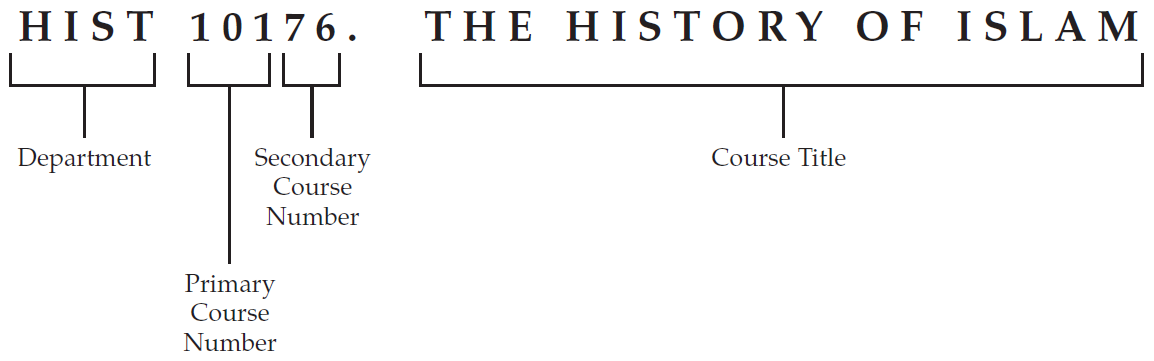Jul 17, 2019
2018-2019 Catalogue
 2018-2019 Updated 2018-2019 Catalogue [ARCHIVED CATALOG] 2016-2017 Catalogue [ARCHIVED CATALOG]
2018-2019 Catalogue [ARCHIVED CATALOG]

# Course Descriptions

## Course Numbering

The College of Wooster uses a five-digit course numbering system. The first three digits indicate the primary course number. The next two digits are the secondary course number and indicate whether there is a special focus for the course. For example:The first letters are the department or program abbreviation. The next three digits are the primary course number (101 is the primary course number for all Introduction to Historial Study courses). The last two digits are the secondary course number. These two digits indicate that the special focus for this HIST 101 course is The History of Islam. A course with a given three-digit primary course number can only be taken once for credit unless specifically indicated otherwise by the department.

The following policy has been used in assigning primary course numbers:

• 100-level courses are usually introductory courses; some 100-level courses do have prerequisites, and students are advised to consult the description for each course.
• 200-level courses are usually beyond the introductory level, although many 200-level courses are open to first-year students and to majors and non-majors.
• 300-level courses are seminars and courses primarily for majors but open to other students with the consent of the instructor.
• The following numbers are for Independent Study: I.S. 40100 (Junior Independent Study), I.S. 45100 and I.S. 45200 (Senior Independent Study).

In addition to the regular course offerings, many departments offer individual tutorials under the number 40000 and internships under 41000. On occasion, departments will offer a course on a special topic as approved by the Educational Policy Committee, designated 19900, 29900, or 39900.

## Abbreviation

In keeping with the general education requirements of the College’s curriculum
(see Degree Requirements ), course listings employ the following abbreviations:

W Writing Intensive

C Studies in Cultural Difference

R Religious Perspectives

Q Quantitative Reasoning

AH Learning Across the Disciplines: Arts and Humanities

HSS Learning Across the Disciplines: History and Social Sciences

MNS Learning Across the Disciplines: Mathematical and Natural Sciences

Except where otherwise noted, all courses carry one course credit.

 Mathematics •  MATH 10000 - Math in Contemporary Society •  MATH 10200 - Introduction to Statistics •  MATH 10400 - Calculus for Social Science •  MATH 10700 - Calculus With Algebra A •  MATH 10800 - Calculus With Algebra B •  MATH 11100 - Calculus & Analytic Geom I •  MATH 11200 - Calculus & Analytic Geom II •  MATH 21100 - Linear Algebra •  MATH 21200 - Multivariate Calculus •  MATH 21500 - Transition to Advanced Mathematics •  MATH 22100 - Differential Equations •  MATH 22300 - Combinatorics & Graph Theory •  MATH 22500 - Mathematical Modeling •  MATH 22700 - Operations Research •  MATH 22900 - Probability and Statistics I •  MATH 27900 - Problem Seminar •  MATH 29901 - Applied Statistical Methods •  MATH 32700 - Numerical Analysis •  MATH 32900 - Probability and Statistics II •  MATH 33000 - Introduction to Topology •  MATH 33200 - Real Analysis I •  MATH 33300 - Real Analysis II •  MATH 33400 - Abstract Algebra •  MATH 33500 - Abstract Algebra II •  MATH 33600 - Functions of a Complex Variable •  MATH 39900 - Special Topics •  MATH 40000 - Tutorial •  MATH 41000 - Math Internships •  MATH 45100 - Independent Study Thesis •  MATH 45200 - Independent Study Thesis